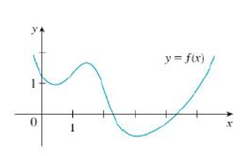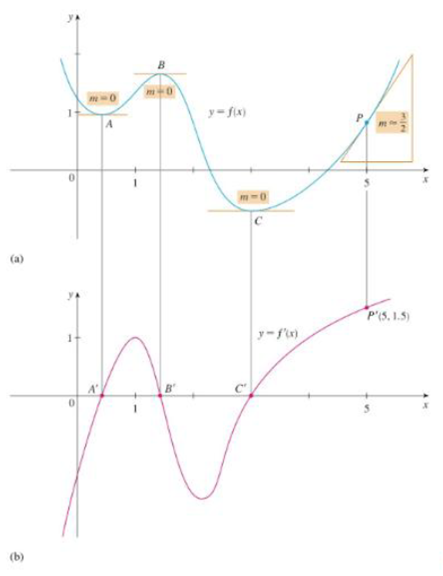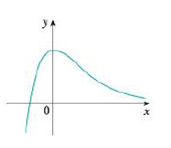Chapter 2.8, Problem 5E### Single Variable Calculus: Early Tr...

8th Edition
James Stewart
ISBN: 9781305270343

#### Solutions

Chapter
Section### Single Variable Calculus: Early Tr...

8th Edition
James Stewart
ISBN: 9781305270343
Textbook Problem

# Trace or copy the graph of the given function .f. (Assume that the axes have equal scales.) Then use the method of Example 1 to sketch the graph of f' below it.Example 1FIGURE 1FIGURE 2To determine

To sketch: The graph of f below the graph of f.

Explanation

From the given graph, it is observed that the graph of f contains the horizontal tangents at one point. Let this point be A.

Note that, the value of the derivative will be zero at the point where the function has the horizontal tangent.

Thus, the graph of f will be zero at the points A.

From the point A to left, the slope of the graph f is strictly positive which implies that the derivative graph f must have functional value in positive

### Still sussing out bartleby?

Check out a sample textbook solution.

See a sample solution

#### The Solution to Your Study Problems

Bartleby provides explanations to thousands of textbook problems written by our experts, many with advanced degrees!

Get Started

#### Solve the equations in Exercises 126. x43x3=0

Finite Mathematics and Applied Calculus (MindTap Course List)

#### Divide: 45.242.4

Elementary Technical Mathematics

#### True or False: If f(x) = g(x) for all x then f(x) = g(x).

Study Guide for Stewart's Single Variable Calculus: Early Transcendentals, 8th

#### Explain the difference between r and .

Introduction To Statistics And Data Analysis